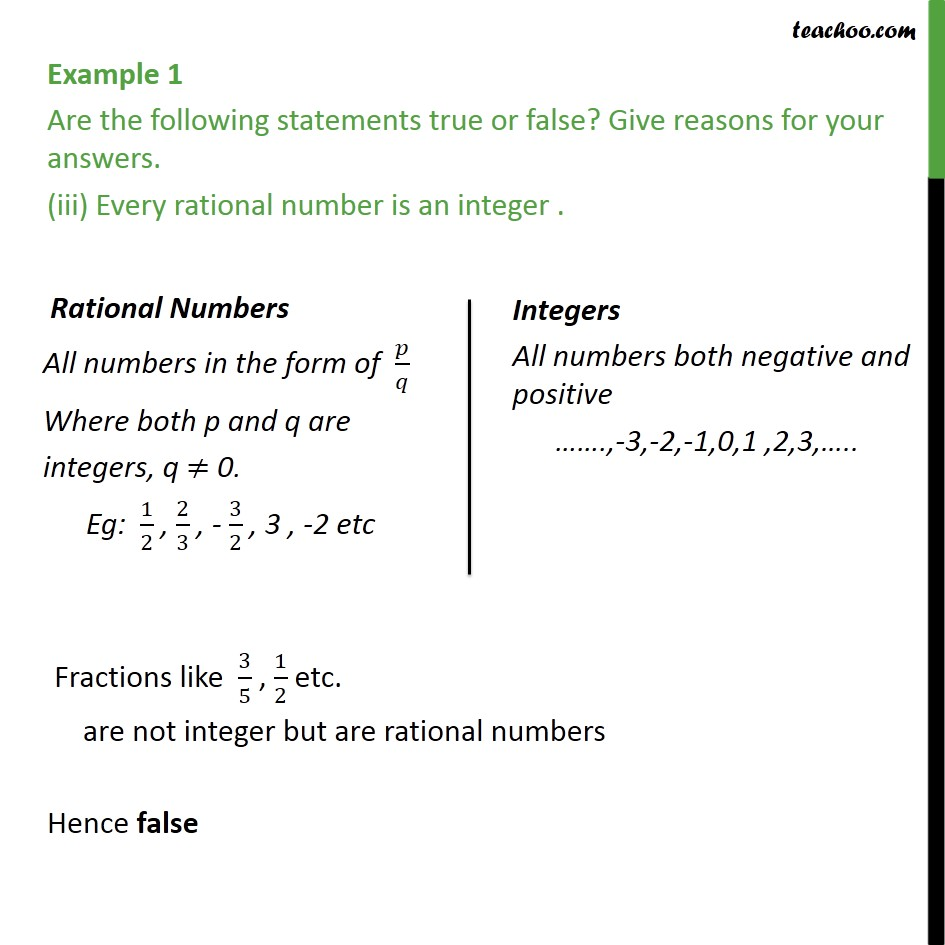1. Chapter 1 Class 9 Number Systems (Term 1)
2. Serial order wise
3. Examples

Transcript

Example 1 Are the following statements true or false? Give reasons for your answers. (iii) Every rational number is an integer . Fractions like 3/5 , 1/2 etc. are not integer but are rational numbers Hence false